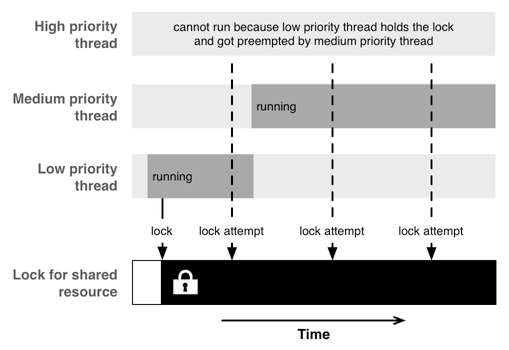OS X 和 iOS 提供了几种不同的 API 来支持并发编程。每一个 API 都具有不同的功能和使用限制，这使它们适合不同的任务。同时，这些 API 处在不同的抽象层级上。我们有可能用其进行非常深入底层的操作，但是这也意味着背负起将任务进行良好处理的巨大责任。

OS X 和 iOS 中的并发编程

线程

uint32_t * inputValues;
size_t count;
};

uint32_t min;
uint32_t max;
};

void * findMinAndMax(void *arg)
{
uint32_t min = UINT32_MAX;
uint32_t max = 0;
for (size_t i = 0; i < info->count; ++i) {
uint32_t v = info->inputValues[i];
min = MIN(min, v);
max = MAX(max, v);
}
free(arg);
result->min = min;
result->max = max;
return result;
}

int main(int argc, const char * argv[])
{
size_t const count = 1000000;
uint32_t inputValues[count];

// 使用随机数字填充 inputValues
for (size_t i = 0; i < count; ++i) {
inputValues[i] = arc4random();
}

// 开始4个寻找最小值和最大值的线程
for (size_t i = 0; i < threadCount; ++i) {
size_t offset = (count / threadCount) * i;
info->inputValues = inputValues + offset;
info->count = MIN(count - offset, count / threadCount);
int err = pthread_create(tid + i, NULL, &findMinAndMax, info);
NSCAssert(err == 0, @"pthread_create() failed: %d", err);
}
// 等待线程退出
for (size_t i = 0; i < threadCount; ++i) {
int err = pthread_join(tid[i], (void **) &(results[i]));
NSCAssert(err == 0, @"pthread_join() failed: %d", err);
}
// 寻找 min 和 max
uint32_t min = UINT32_MAX;
uint32_t max = 0;
for (size_t i = 0; i < threadCount; ++i) {
min = MIN(min, results[i]->min);
max = MAX(max, results[i]->max);
free(results[i]);
results[i] = NULL;
}

NSLog(@"min = %u", min);
NSLog(@"max = %u", max);
return 0;
}

@property (nonatomic) NSUInteger min;
@property (nonatomic) NSUInteger max;
- (instancetype)initWithNumbers:(NSArray *)numbers;
@end

NSArray *_numbers;
}

- (instancetype)initWithNumbers:(NSArray *)numbers
{
self = [super init];
if (self) {
_numbers = numbers;
}
return self;
}

- (void)main
{
NSUInteger min;
NSUInteger max;
// 进行相关数据的处理
self.min = min;
self.max = max;
}
@end

NSUInteger numberCount = self.numbers.count;
for (NSUInteger i = 0; i < threadCount; i++) {
NSUInteger offset = (count / threadCount) * i;
NSUInteger count = MIN(numberCount - offset, numberCount / threadCount);
NSRange range = NSMakeRange(offset, count);
NSArray *subset = [self.numbers subarrayWithRange:range];
}

Grand Central Dispatch

GCD 带来的另一个重要改变是，作为开发者可以将工作考虑为一个队列，而不是一堆线程，这种并行的抽象模型更容易掌握和使用。

GCD 公开有 5 个不同的队列：运行在主线程中的 main queue，3 个不同优先级的后台队列，以及一个优先级更低的后台队列（用于 I/O）。 另外，开发者可以创建自定义队列：串行或者并行队列。自定义队列非常强大，在自定义队列中被调度的所有 block 最终都将被放入到系统的全局队列中和线程池中。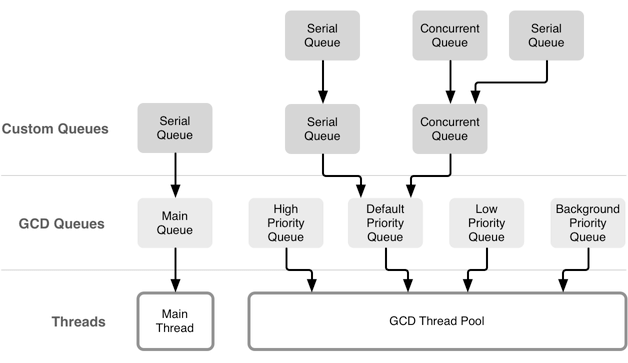Operation Queues

NSOperationQueue 有两种不同类型的队列：主队列和自定义队列。主队列运行在主线程之上，而自定义队列在后台执行。在两种类型中，这些队列所处理的任务都使用 NSOperation 的子类来表述。

@implementation YourOperation
- (void)main
{
// 进行处理 ...
}
@end

@implementation YourOperation
- (void)start
{
self.isExecuting = YES;
self.isFinished = NO;
// 开始处理，在结束时应该调用 finished ...
}

- (void)finished
{
self.isExecuting = NO;
self.isFinished = YES;
}
@end

- (void)main
{
while (notDone && !self.isCancelled) {
// 进行处理
}
}

NSOperationQueue *queue = [[NSOperationQueue alloc] init];
YourOperation *operation = [[YourOperation alloc] init];

// 代码...
}];

Run Loops

Run loop 比起操作队列或者 GCD 来说容易使用得多，因为通过 run loop ，你不必处理并发中的复杂情况，就能异步地执行任务。

并发编程中面临的挑战

资源共享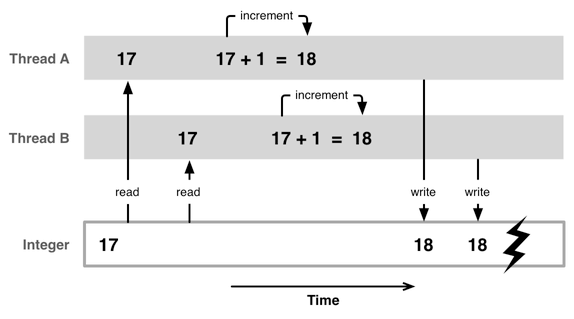互斥锁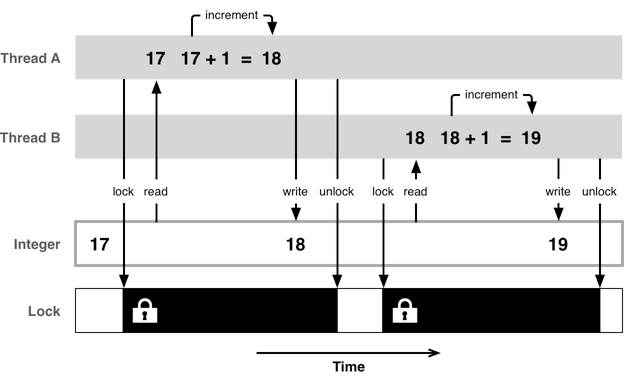死锁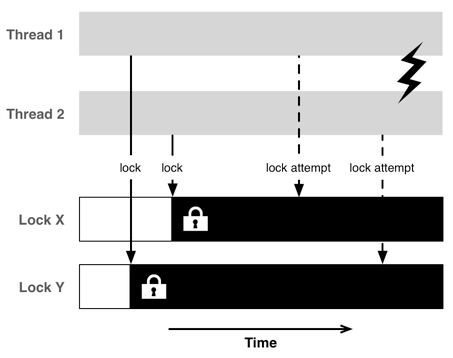void swap(A, B)
{
lock(lockA);
lock(lockB);
int a = A;
int b = B;
A = b;
B = a;
unlock(lockB);
unlock(lockA);
}

swap(X, Y); // 线程 1
swap(Y, X); // 线程 2

优先级反转